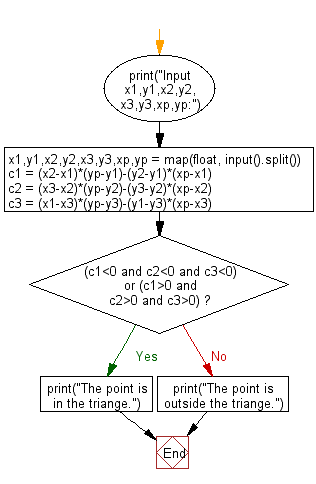﻿ Python: Check whether a point (x,y) is in a triangle or not - w3resource# Python: Check whether a point (x,y) is in a triangle or not

## Python Basic - 1: Exercise-40 with Solution

Write a Python program to check whether a point (x,y) is in a triangle or not. There is a triangle formed by three points.

Input:
x1,y1,x2,y2,x3,y3,xp,yp separated by a single space.

Sample Solution:

Python Code:

``````print("Input x1,y1,x2,y2,x3,y3,xp,yp:")
x1,y1,x2,y2,x3,y3,xp,yp = map(float, input().split())
c1 = (x2-x1)*(yp-y1)-(y2-y1)*(xp-x1)
c2 = (x3-x2)*(yp-y2)-(y3-y2)*(xp-x2)
c3 = (x1-x3)*(yp-y3)-(y1-y3)*(xp-x3)
if (c1<0 and c2<0 and c3<0) or (c1>0 and c2>0 and c3>0):
print("The point is in the triangle.")
else:
print("The point is outside the triangle.")
``````

Sample Output:

```Input x1,y1,x2,y2,x3,y3,xp,yp:
2 3 4 5 6 8 7 1
The point is outside the triangle.
```

Flowchart:Python Code Editor:

Have another way to solve this solution? Contribute your code (and comments) through Disqus.

What is the difficulty level of this exercise?

Test your Programming skills with w3resource's quiz.

﻿

## Python: Tips of the Day

Iterating over dictionaries using 'for' loops:

I am a bit puzzled by the following code: d = {'x': 1, 'y': 2, 'z': 3} for key in d: print key, 'corresponds to', d[key] What I don't understand is the key portion. How does Python recognize ...

key is just a variable name.

```for key in d:
```

For Python 3.x:

```for key, value in d.items():
```

For Python 2.x:

```for key, value in d.iteritems():
```

To test for yourself, change the word key to poop.

In Python 3.x, iteritems() was replaced with simply items(), which returns a set-like view backed by the dict, like iteritems() but even better. This is also available in 2.7 as viewitems().

The operation items() will work for both 2 and 3, but in 2 it will return a list of the dictionary's (key, value) pairs, which will not reflect changes to the dict that happen after the items() call. If you want the 2.x behavior in 3.x, you can call list(d.items()).

Ref: https://bit.ly/37dm0Qo